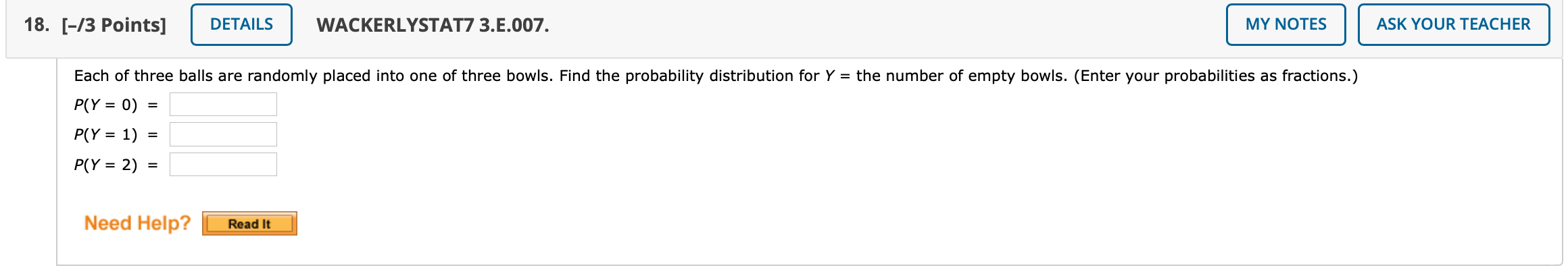# Question Solved1 Answer18. [-13 Points] DETAILS WACKERLYSTAT7 3.E.007. MY NOTES ASK YOUR TEACHER Each of three balls are randomly placed into one of three bowls. Find the probability distribution for Y = the number of empty bowls. (Enter your probabilities as fractions.) P(Y = 0) = P(Y = 1) P(Y = 2) = Need Help? Read ItXVDE9Z The Asker · Probability and StatisticsTranscribed Image Text: 18. [-13 Points] DETAILS WACKERLYSTAT7 3.E.007. MY NOTES ASK YOUR TEACHER Each of three balls are randomly placed into one of three bowls. Find the probability distribution for Y = the number of empty bowls. (Enter your probabilities as fractions.) P(Y = 0) = P(Y = 1) P(Y = 2) = Need Help? Read It
More
Transcribed Image Text: 18. [-13 Points] DETAILS WACKERLYSTAT7 3.E.007. MY NOTES ASK YOUR TEACHER Each of three balls are randomly placed into one of three bowls. Find the probability distribution for Y = the number of empty bowls. (Enter your probabilities as fractions.) P(Y = 0) = P(Y = 1) P(Y = 2) = Need Help? Read It